Integrals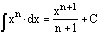The integral of a power is the reciprocal of the exponent plus one times x to the power of the exponnent plus one.The integral of a sum is the sum of the integrals of each function being added.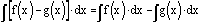The integral of a difference is the difference of the integrals of the functions being subtracted.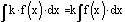The integral of a constant times a function is the constant times the integral of the function.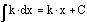The integral of a constant is the constant times x.The integral of an exponential function is the product of the exponential function and the reciprocal of the natural logarithm of the base.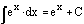Since the natural logarithm of e is 1, the integral of e^(x) is e^(x).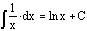The integral of 1 divided by x is the natural logarithm of x.The integral of the sine of x is the opposite of the cosine of x.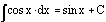The integral of the cosine of x is the sine of x.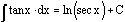The integral of the tangent of x is the natural logarithm of the secant of x.The integral of the secant of x is the natural logarithm of the sum of the secant of x and the tangent of x.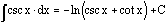The integral of the cosecant of x is the opposite of the natural logarithm of the sum of the cosecant of x and the cotangent of x.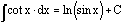The integral of the cotangent of x is the natural logarithm of the sine of x.

Back to Formula